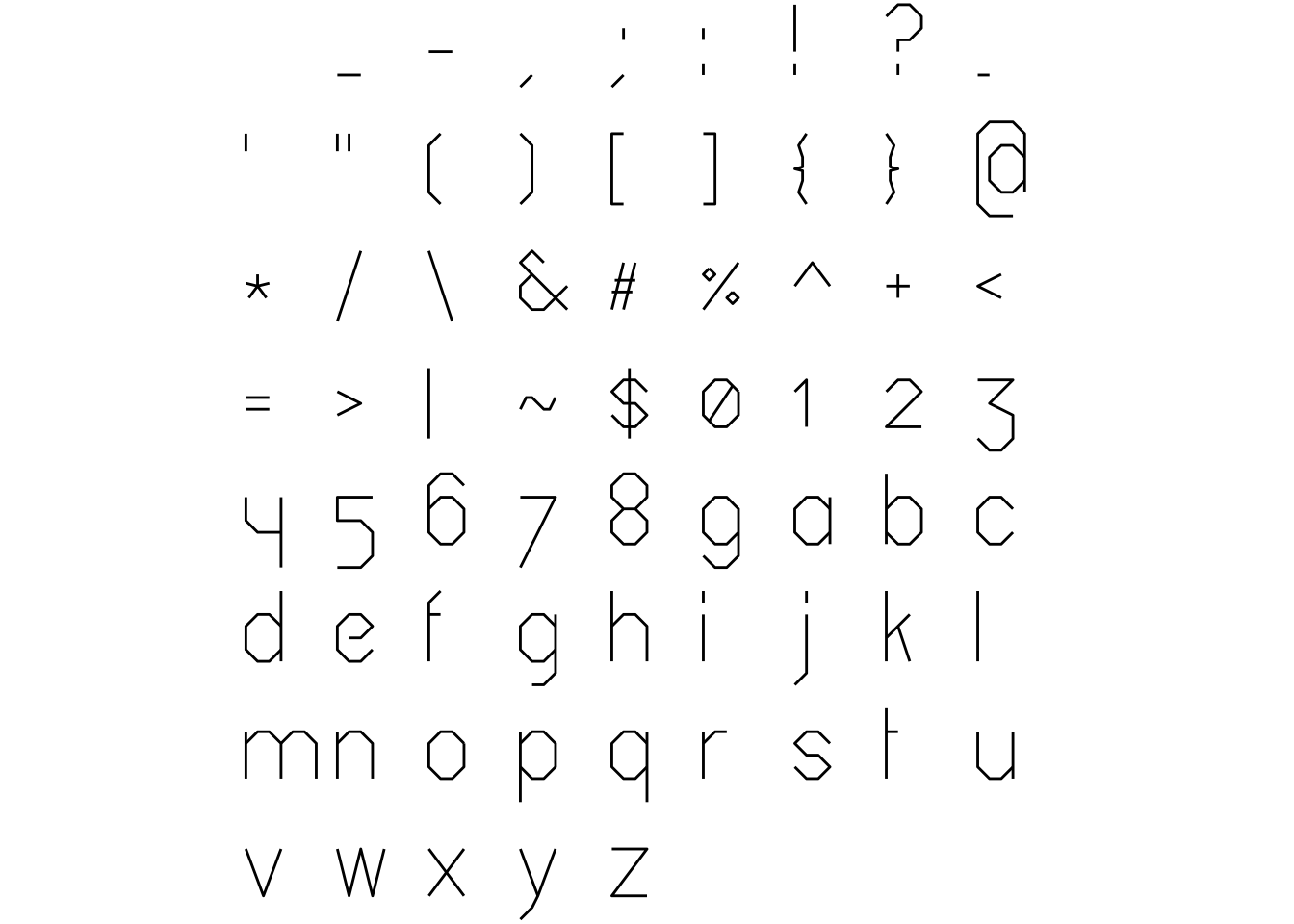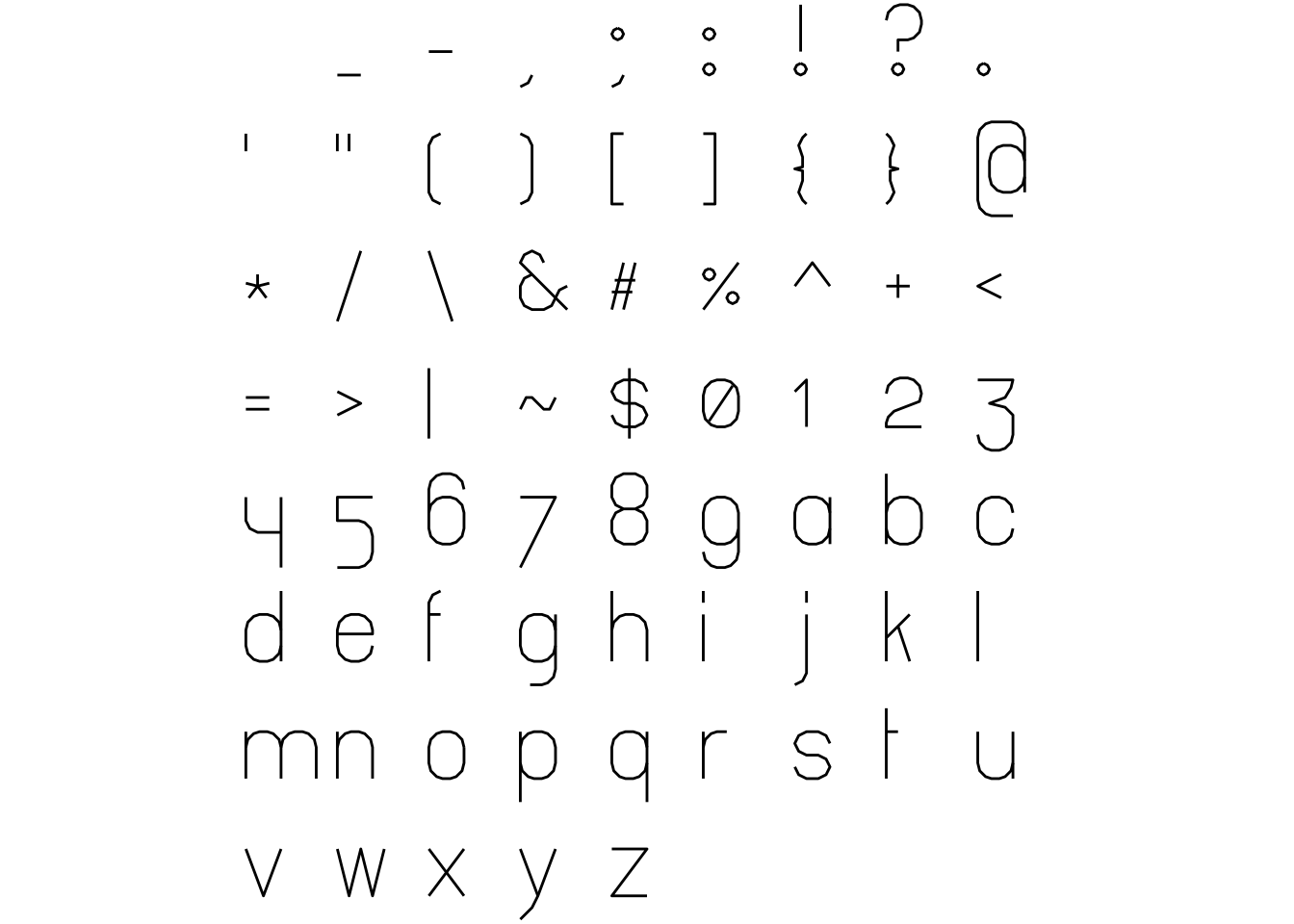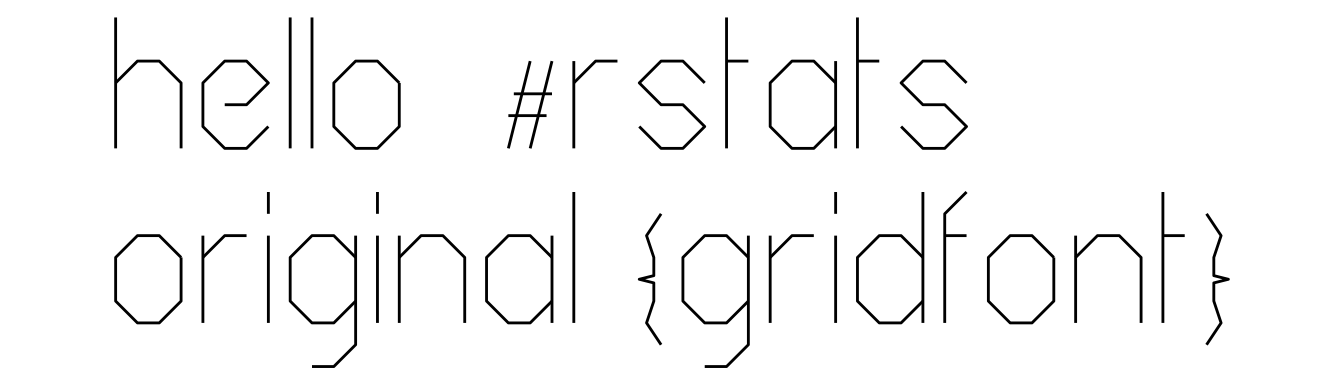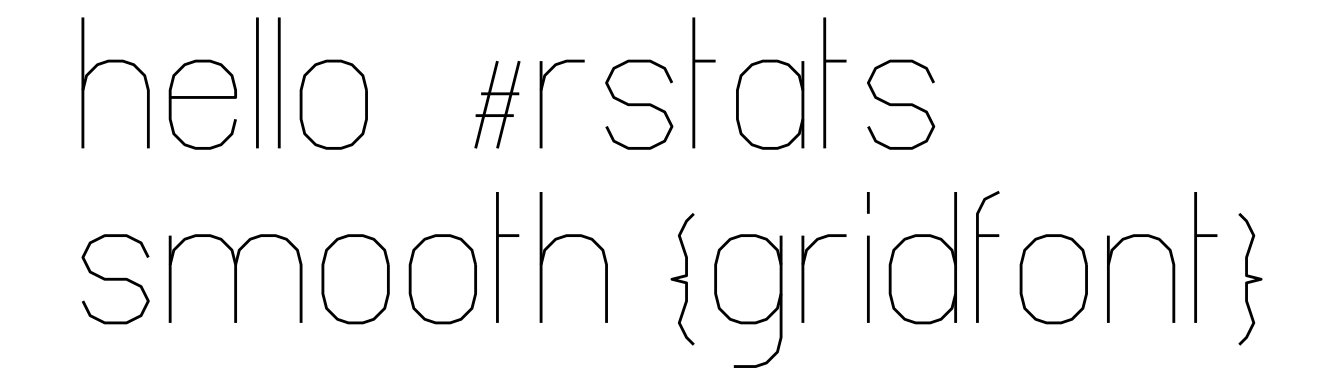# gridfontAn R repackaging of the gridfont vector font created by inconvergent.

This package is similar to the packages hershey and arcadefont in that it provides point and stroke information, but how this is actually rendered is up to you.

In the examples below ggplot is used to render the strokes for each glyph as geom_path objects.

#### What’s in the box?

• original_df - a data.frame of coordinates and stroke information for all the provided glyphs for the original version of the font.
• smooth_df - a data.frame of coordinates and stroke information for all the provided glyphs for the smooth version of the font.
• create_text_df() - a function to create stroke information for all the characters in a given string, and offset them appropriately (vertically and horizontally) so that they are ready to render.
• original_json, smooth_json the original JSON data read in using jsonlite and provided as list objects.

#### Installation

You can install from github with:

# install.packages('remotes')
remotes::install_github("coolbutuseless/gridfont")

This package is made available under the MIT license. See the file “LICENCSE”.

The original font data was made available by Anders Hoff (a.k.a Inconvergent) also under the MIT license. See the file “LICENSE-font”

## Sample sheet for the ‘original’ font

ggplot(gridfont::original_df, aes(x, y)) +
geom_path(aes(group = as.factor(stroke)), na.rm=TRUE) +
coord_equal() +
theme_void() +
facet_wrap(~char)  +
theme(
strip.background = element_blank(),
strip.text.x     = element_blank()
)## Sample sheet for the ‘smooth’ font

ggplot(gridfont::smooth_df, aes(x, y)) +
# geom_point() +
geom_path(aes(group = as.factor(stroke)), na.rm=TRUE) +
coord_equal() +
theme_void() +
facet_wrap(~char)  +
theme(
strip.background = element_blank(),
strip.text.x     = element_blank()
)## Creating blocks of text

gridfont includes create_text_df() as an easy way to create stroke data for all the characters from a given string.

text    <- 'hello  #RStats\noriginal {gridfont}'
plot_df <- create_text_df(text, font='original')

ggplot(plot_df, aes(x, y)) +
geom_path(aes(group = interaction(char_idx, stroke)), na.rm=TRUE) +
coord_equal() +
theme_void()text    <- 'hello  #RStats\nsmooth {gridfont}'
plot_df <- create_text_df(text, font='smooth')

ggplot(plot_df, aes(x, y)) +
geom_path(aes(group = interaction(char_idx, stroke)), na.rm=TRUE) +
coord_equal() +
theme_void()## Animated rendering

Now that all the stroke information is available, we can render each stroke, character-by-character to get a “writing” effect.